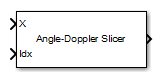Data Cube Slicer

Slice a data cube along specified dimensions

Library

phasedstaplib

•Description

The Data Cube Slicer block slices a data cube along the specified dimensions. The input is a data cube of dimensions M-by-Q-by-N. The first dimension is range, or fast time. The second dimension is angle, or channels. The third dimension is Doppler, or slow time. If you set Output Slice to Angle-Doppler, the output has dimension Q-by-N. If you set Output Slice to Range-Doppler, the output has dimension M-by-N. If you set Output Slice to Range-angle, the output has dimension M-by-Q.

Parameters

Output slice

Select desired output for a M-by-Q-by-N data cube. Parameter values are

ValueDimension
Angle-DopplerQ-by-N
Range-DopplerM-by-N
Range-angleM-by-Q

Ports

PortSupported Data Types
XDouble-precision floating point
IdxDouble-precision floating point
OutDouble-precision floating point

Extended Capabilities

C/C++ Code GenerationGenerate C and C++ code using Simulink® Coder™.

Introduced in R2014b